Bergman-Shilov boundary

(Redirected from Bergman–Shilov boundary)

The concept of the Bergman–Shilov boundary is credited to both S. Bergman and G.E. Shilov. Bergman, in 1931, introduced the concept of a distinguished boundary in connection with his studies of holomorphic functions of two complex variables [a1]. He discovered that, for a large class of domains, a holomorphic function of two or more variables is completely determined by its values on a relatively small closed subset of the topological boundary of the domain. This phenomenon does not occur in function theory of one complex variable (cf. also Analytic function). On the other hand, in the 1940s Shilov introduced the concept of a minimal boundary, now called the Shilov boundary, in connection with his studies on commutative Banach algebras (cf. also Boundary (in the theory of uniform algebras); Commutative Banach algebra). Much of the content of this work can be found in [a3].

In modern terminology, the Shilov boundary is defined as follows: Letbe a compact space and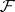an algebra of continuous complex-valued functions onwhich separates the points of(cf. Algebra of functions). A boundary foris a closed subsetofsuch that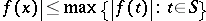for alland. The Shilov boundary offor, denoted by, is defined as the intersection of all boundariesoffor. It was proved by Shilov [a3] that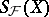is non-empty and is a boundary for.

Ifis a bounded domain in an-dimensional complex space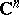,, and if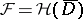is the set of all functionsthat are holomorphic on(the closure of), then the Shilov boundary of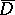foris called the Bergman–Shilov boundary of, usually denoted by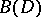. By the maximum principle,is always a subset of the topological boundary of. However, when, for many domainsis a proper subset of the boundary of. For example, if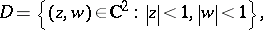then, which is a two-dimensional closed subset of the three-dimensional boundary of. This is the case for a wide class of domains, including all analytic polyhedra (cf. Analytic polyhedron). On the other hand, ifthenis equal to the boundary of. More generally, ifis strictly pseudo-convex, that is,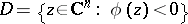, whereis a plurisubharmonic function in a neighbourhood of(cf. also Pseudo-convex and pseudo-concave), thenis equal to the topological boundary of. A fairly comprehensive treatment of the Shilov and Bergman–Shilov boundaries can be found in [a2].